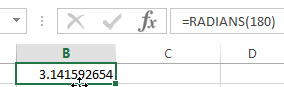# ExcelHow

This post will guide you how to use Excel RADIANS function with syntax and examples in Microsoft excel.

### Description

The RADIANS function is a build-in function in Microsoft Excel and it is categorized as a Math and Trigonometry Function.

The RADIANS function is available in Excel 2016, Excel 2013, Excel 2010, Excel 2007, Excel 2003, Excel XP, Excel 2000, Excel 2011 for Mac.

### Syntax

The syntax of the RADIANS function is as below:

`=RADIANS (angle)`

Where the RADIANS function arguments is:

• angle –This is a required argument. Any angle in degrees that you wan to convert to radians.

The below examples will show you how to use Excel RADIANS Function to convert degrees to radians.

1# to convert 180 degrees as radians, enter the following formula in Cell B1.